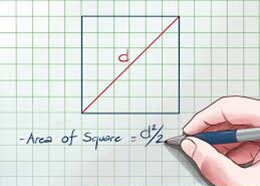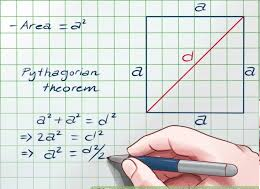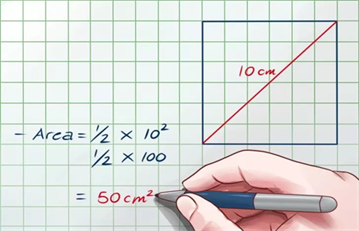# Area Of Square Using DiagonalsApart from the traditional method of finding the area of the square by squaring the sides, there’s one of the most innovative methods to compute the area of a square.

It’s not always mandatory to have the measure of the side for computing a square’s area. If we have the length of the diagonal then the area can be calculated asNow, you must be wondering how this formula came into the picture, So, to remove any kind of doubt here’s given the derivation of the concept using the Pythagoras theorem as shown below in the figure:In the above figure, the square of side ‘a’ unit, has been divided into two right triangles with the help of diagonal of length d units.
We thus obtain two isosceles right triangles each with base equal to height equal to ‘a’ units and the hypotenuse as d units.

On applying the Pythagoras theorem and equating it with the square of the diagonal and further solving. Eventually, we obtain the value a² = d²/2 which is nothing but the area of the square using the diagonal concept.

An example is shown below for deducing the area of the square by the diagonal of length 10 cm:Applying the diagonal formula for the area of the square (as obtained by the Pythagorean concept) we get the area equal to 50 square cms.

Apart from the above-explained concepts, there are several other interesting ways to calculate the square area, perimeter, volumes and other geometrical concepts. Learn more with BYJU’S- The Learning App.